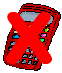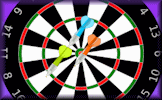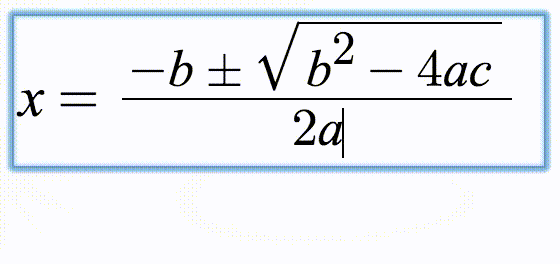# Dr Tim's Indices Challenge

## Dr Tim Honeywill has come up with these challenges to test your understanding of indices and algebra.

##### IndicesLevel 1Level 2Exam-StyleDescriptionHelpMore IndicesThis is level 1: Dr Tim's tricky tasks. You can earn a trophy if you get at least 7 questions correct and you do this activity online.

The letters (variables) in your answers should be in alphabetical order with the constant term (if any) at the end.

 1. If $$2^n=2^p \times 2^q$$,write down an expression for $$n$$ in terms of $$p$$ and $$q$$. n=2. If $$2^n= 2^p \times 2^q \times 2$$,write down an expression for $$n$$ in terms of $$p$$ and $$q$$. n=3. If $$2^n= 2^p \times 2^q \times 8$$,write down an expression for $$n$$ in terms of $$p$$ and $$q$$. n=4. If $$2^n= \frac {2^p \times 2^q}{2}$$,write down an expression for $$n$$ in terms of $$p$$ and $$q$$. n=5. If $$2^n=4^p \times 8^q \times 16$$,write down an expression for $$n$$ in terms of $$p$$ and $$q$$. n=6. If $$2^n= \frac{2 \times 4^p}{4 \times 2^q}$$,write down an expression for $$n$$ in terms of $$p$$ and $$q$$. n=7. If $$2^n=4^p \times 8^n$$,write down an expression for $$n$$ in terms of $$p$$. n=8. If $$2^n=(2 \times 4^p )^2 \times (8 \times 16^q )^3$$,write down an expression for $$n$$ in terms of $$p$$ and $$q$$. n=9. If $$3^n=9^p \times 27$$,write down an expression for $$n$$ in terms of $$p$$. n=10. If $$3 \times 3^n= 9 \times 9^p \times 27 \times 27^q$$,write down an expression for $$n$$ in terms of $$p$$ and $$q$$. n=Check

This is Dr Tim's Indices Challenge level 1. You can also try:
Level 2

## Instructions

Try your best to answer the questions above. Type your answers into the boxes provided leaving no spaces. As you work through the exercise regularly click the "check" button. If you have any wrong answers, do your best to do corrections but if there is anything you don't understand, please ask your teacher for help.

When you have got all of the questions correct you may want to print out this page and paste it into your exercise book. If you keep your work in an ePortfolio you could take a screen shot of your answers and paste that into your Maths file.

## Transum.org

This web site contains over a thousand free mathematical activities for teachers and pupils. Click here to go to the main page which links to all of the resources available.## More Activities:

Mathematicians are not the people who find Maths easy; they are the people who enjoy how mystifying, puzzling and hard it is. Are you a mathematician?

Comment recorded on the 1 February 'Starter of the Day' page by M Chant, Chase Lane School Harwich:

"My year five children look forward to their daily challenge and enjoy the problems as much as I do. A great resource - thanks a million."

Comment recorded on the 2 May 'Starter of the Day' page by Angela Lowry, :

"I think these are great! So useful and handy, the children love them.
Could we have some on angles too please?"

Each month a newsletter is published containing details of the new additions to the Transum website and a new puzzle of the month.

The newsletter is then duplicated as a podcast which is available on the major delivery networks. You can listen to the podcast while you are commuting, exercising or relaxing.

Transum breaking news is available on Twitter @Transum and if that's not enough there is also a Transum Facebook page.

#### Digital DartsAn online darts game for one or two players requiring skill, strategy and mental arithmetic. It provides an enjoyable way to practise adding and subtracting two and three digit numbers.

There are answers to this exercise but they are available in this space to teachers, tutors and parents who have logged in to their Transum subscription on this computer.

A Transum subscription unlocks the answers to the online exercises, quizzes and puzzles. It also provides the teacher with access to quality external links on each of the Transum Topic pages and the facility to add to the collection themselves.

Subscribers can manage class lists, lesson plans and assessment data in the Class Admin application and have access to reports of the Transum Trophies earned by class members.

Subscribe

## Go Maths

Learning and understanding Mathematics, at every level, requires learner engagement. Mathematics is not a spectator sport. Sometimes traditional teaching fails to actively involve students. One way to address the problem is through the use of interactive activities and this web site provides many of those. The Go Maths page is an alphabetical list of free activities designed for students in Secondary/High school.

## Maths Map

Are you looking for something specific? An exercise to supplement the topic you are studying at school at the moment perhaps. Navigate using our Maths Map to find exercises, puzzles and Maths lesson starters grouped by topic.

## Teachers

If you found this activity useful don't forget to record it in your scheme of work or learning management system. The short URL, ready to be copied and pasted, is as follows:

Alternatively, if you use Google Classroom, all you have to do is click on the green icon below in order to add this activity to one of your classes.

It may be worth remembering that if Transum.org should go offline for whatever reason, there are mirror sites at Transum.com and Transum.info that contain most of the resources that are available here on Transum.org.

When planning to use technology in your lesson always have a plan B!

Do you have any comments? It is always useful to receive feedback and helps make this free resource even more useful for those learning Mathematics anywhere in the world. Click here to enter your comments.For All:

Questions written by Dr Tim Honeywill (@HoneywillTim)

Background photograph by Yvonne Brown

Scan the QR code below to visit the online version of this activity.https://Transum.org/go/?Num=857

## Description of Levels

CloseIndices - The traditional, easier indices questions.

Level 1 - Dr Tim's tricky tasks

Level 2 - Dr Tim's tortuous tasks

Exam Style Questions - A collection of problems in the style of GCSE or IB/A-level exam paper questions (worked solutions are available for Transum subscribers).

More on this topic including lesson Starters, visual aids, investigations and self-marking exercises.

Answers to this exercise are available lower down this page when you are logged in to your Transum account. If you don’t yet have a Transum subscription one can be very quickly set up if you are a teacher, tutor or parent.

## Curriculum Reference

See the National Curriculum page for links to related online activities and resources.

## Reminders

 $$5^1=5$$ $$5^0=1$$ $$5^{-1}=\frac15$$ $$5^a \times 5^b = 5^{a+b}$$ $$5^a \div 5^b = 5^{a-b}$$ $$(5^a)^b = 5^{ab}$$

The answers, particularly at level 2, can be written a variety of different forms even within the 'alphabetical order' constraint. If you get an answer marked wrong but are convinced it is correct try writing it in a different format.

Don't wait until you have finished the exercise before you click on the 'Check' button. Click it often as you work through the questions to see if you are answering them correctly. You can double-click the 'Check' button to make it float at the bottom of your screen.

Answers to this exercise are available lower down this page when you are logged in to your Transum account. If you don’t yet have a Transum subscription one can be very quickly set up if you are a teacher, tutor or parent.

### Typing Mathematical Notation

These exercises use MathQuill, a web formula editor designed to make typing Maths easy and beautiful. Watch the animation below to see how common mathematical notation can be created using your keyboard.Close